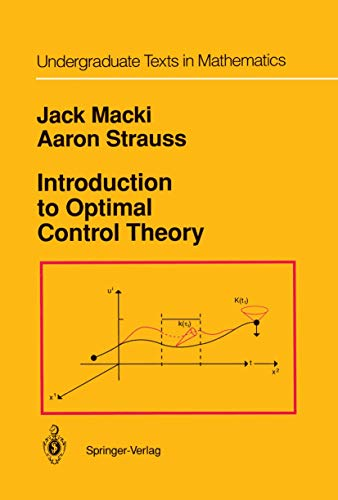• Free Shipping on all orders in Australia
• Over 7 million books in stock
• Proud to be B-Corp
• We aim to be carbon neutral by 2022
• Over 120,000 Trustpilot reviews
Item 1 of 0# Introduction to Optimal Control Theory by Jack Macki

Condition - New
\$117.59
Only 2 left

## Summary

We have tried to keep two types of reader in mind: (1) mathematicians, graduate students, and advanced undergraduates in mathematics who want a concise introduction to a field which contains nontrivial interesting applications of mathematics (for example, weak convergence, convexity, and the theory of ordinary differential equations);

## Introduction to Optimal Control Theory Summary

### Introduction to Optimal Control Theory by Jack Macki

This monograph is an introduction to optimal control theory for systems governed by vector ordinary differential equations. It is not intended as a state-of-the-art handbook for researchers. We have tried to keep two types of reader in mind: (1) mathematicians, graduate students, and advanced undergraduates in mathematics who want a concise introduction to a field which contains nontrivial interesting applications of mathematics (for example, weak convergence, convexity, and the theory of ordinary differential equations); (2) economists, applied scientists, and engineers who want to understand some of the mathematical foundations. of optimal control theory. In general, we have emphasized motivation and explanation, avoiding the "definition-axiom-theorem-proof" approach. We make use of a large number of examples, especially one simple canonical example which we carry through the entire book. In proving theorems, we often just prove the simplest case, then state the more general results which can be proved. Many of the more difficult topics are discussed in the "Notes" sections at the end of chapters and several major proofs are in the Appendices. We feel that a solid understanding of basic facts is best attained by at first avoiding excessive generality. We have not tried to give an exhaustive list of references, preferring to refer the reader to existing books or papers with extensive bibliographies. References are given by author's name and the year of publication, e.g., Waltman .

I Introduction and Motivation.- 1 Basic Concepts.- 2 Mathematical Formulation of the Control Problem.- 3 Controllability.- 4 Optimal Control.- 5 The Rocket Car.- Exercises.- Notes.- II Controllability.- 1 Introduction: Some Simple General Results.- 2 The Linear Case.- 3 Controllability for Nonlinear Autonomous Systems.- 4 Special Controls.- Exercises.- Appendix: Proof of the Bang-Bang Principle.- III Linear Autonomous Time-Optimal Control Problems.- 1 Introduction: Summary of Results.- 2 The Existence of a Time-Optimal Control; Extremal Controls; the Bang-Bang Principle.- 3 Normality and the Uniqueness of the Optimal Control.- 4 Applications.- 5 The Converse of the Maximum Principle.- 6 Extensions to More General Problems.- Exercises.- IV Existence Theorems for Optimal Control Problems.- 1 Introduction.- 2 Three Discouraging Examples. An Outline of the Basic Approach to Existence Proofs.- 3 Existence for Special Control Classes.- 4 Existence Theorems under Convexity Assumptions.- 5 Existence for Systems Linear in the State.- 6 Applications.- Exercises.- Notes.- V Necessary Conditions for Optimal Controls-The Pontryagin Maximum Principle.- 1 Introduction.- 2 The Pontryagin Maximum Principle for Autonomous Systems.- 3 Applying the Maximum Principle.- 4 A Dynamic Programming Approach to the Proof of the Maximum Principle.- 5 The PMP for More Complicated Problems.- Exercises.- Appendix to Chapter V-A Proof of the Pontryagin Maximum Principle.- Mathematical Appendix.

NLS9781461256731
9781461256731
1461256739
Introduction to Optimal Control Theory by Jack Macki
New
Paperback
Springer-Verlag New York Inc.
2011-10-20
168
N/A
Book picture is for illustrative purposes only, actual binding, cover or edition may vary.
This is a new book - be the first to read this copy. With untouched pages and a perfect binding, your brand new copy is ready to be opened for the first time